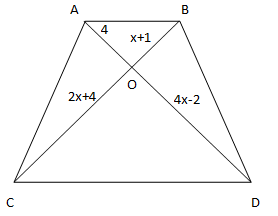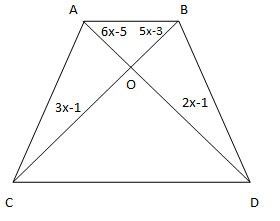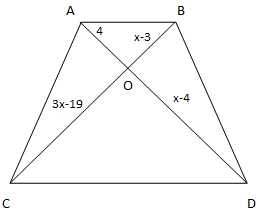# Class 10 RD Sharma Solutions – Chapter 4 Triangles – Exercise 4.4

• Last Updated : 07 Apr, 2021

### Question 1. (i) In fig., if AB || CD, find the value of x.

Solution:Attention reader! All those who say programming isn't for kids, just haven't met the right mentors yet. Join the  Demo Class for First Step to Coding Coursespecifically designed for students of class 8 to 12.

The students will get to learn more about the world of programming in these free classes which will definitely help them in making a wise career choice in the future.

Given,

AB∥ CD.

To find the value of x.

Now,

AO/ CO = BO/ DO                         [Diagonals of a parallelogram bisect each other]

⇒ 4/ (4x – 2) = (x +1)/ (2x + 4)

4(2x + 4) = (4x – 2)(x +1)

8x + 16 = x(4x – 2) + 1(4x – 2)

8x + 16 = 4x2 – 2x + 4x – 2

-4x2 + 8x + 16 + 2 – 2x = 0

-4x2 + 6x + 8 = 0

4x2 – 6x – 18 = 0

4x2 – 12x + 6x – 18 = 0

4x(x – 3) + 6(x – 3) = 0

(4x + 6) (x – 3) = 0

∴ x = – 6/4 or x = 3

### (ii) In fig., if AB || CD, find the value of x.

Solution:Given,

AB∥ CD.

To find the value of x.

Now,

AO/ CO = BO/ DO                     [Diagonals of a parallelogram bisect each other]

⇒ (6x – 5)/ (2x + 1) = (5x – 3)/ (3x – 1)

(6x – 5)(3x – 1) = (2x + 1)(5x – 3)

3x(6x – 5) – 1(6x – 5) = 2x(5x – 3) + 1(5x – 3)

18x2 – 10x2 – 21x + 5 + x +3 = 0

8x2 – 20x + 8 = 0

8x2 – 16x – 4x + 8 = 0

8x(x – 2) – 4(x – 2) = 0

(8x – 4)(x – 2) = 0

x = 4/8 = 1/2 or x = -2

∴ x= 1/2

### (iii) In fig., if AB || CD. If OA = 3x – 19, OB = x – 4, OC = x- 3 and OD = 4, find x.

Solution:Given,

AB∥ CD.

OA = 3x – 19, OB = x – 4, OC = x- 3 and OD = 4

To find the value of x.

Now,

AO/ CO = BO/ DO                      [Diagonals of a parallelogram bisect each other]

(3x – 19)/ (x – 3) = (x–4)/ 4

4(3x – 19) = (x – 3) (x – 4)

12x – 76 = x(x – 4) -3(x – 4)

12x – 76 = x2 – 4x – 3x + 12

-x2 + 7x – 12 + 12x -76 = 0

-x2 + 19x – 88 = 0

x2 – 19x + 88 = 0

x2 – 11x – 8x + 88 = 0

x(x – 11) – 8(x – 11) = 0

∴ x = 11 or x = 8

My Personal Notes arrow_drop_up